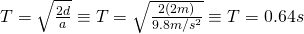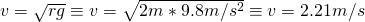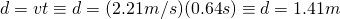# Caclulating distance related to tangential velocity

A question was posed in Physics as follows:

A 60 kg ball is tied to the end of a 50 cm long mass-less string and swung in a vertical circle. The center of the circle is 150 cm above the floor. The ball is swung at the minimum speed necessary to make it over the top without the string going slack. If the string is released at the instant the ball is at the top of the loop, how far to the right (in meters) does the ball hit the ground?

Okay, now we need to look up the key items in this question:

mass = 60 kg
radius = .5 m (50 cm)
height = 1.5 m (150 cm)
gravitational acceleration = 9.8 m/s^2
vertical circle (tossed overhead with release at the peak)
minimum speed for no slack ( t must be greater than or equal to 0)

Now, what this tells us is that we need to a) find the tangential velocity at the top of the circle, b) find the minimum tension on the rope that it won’t go slack at the top, c) the time that it takes the ball to fall back to the ground from it’s highest point (this will allow us to calculate distance on the x-axis).  It is important to note that for this equation mass is extra information.

Let’s solve for the time from the top of the circle to the ground since that’s the easiest to get right away (a quick note, the peak is the height to the middle plus the radius of the circle, so, 2 meters):Conceptually we need to realize that the velocity of the object at the top of the circle will be related to the gravity, radius and tension.  Since we want the minimum speed possible, that means the tension must be 0 or greater but not less than 0.  That means that the tension goes out of the problem because we’re just dealing with gravity and the radius of the circle since tension is 0.  That means we can figure out velocity based on the relationship between gravity and the radius of the circle:Now that we have the velocity and the time that it takes for the object to get to the ground we use the very simple formula d = vt in order to find the distance the object travels on the x-axis.Ta-da!  Hopefully this is somewhat helpful in explaining how to get this answer.  Please feel free to comment and or correct me if you find errors.